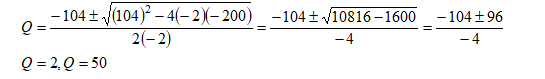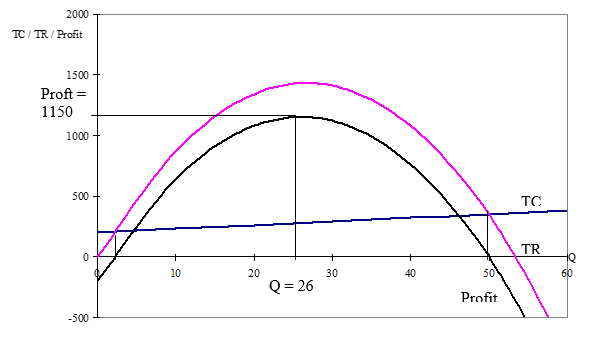In: Economics

# A firms demand function for a good is given by P = 107-2Q and their total cost function is given by

A firms demand function for a good is given by P = 107-2Q and their total cost function is given by TC = 200+3Q .

i). Obtain an expression for total revenue profit in terms of Q

ii).  For what values of Q does the firm break even.

iii). llustrate the answer to (ii) using sketches of the total cost function, the total revenue function and the profit function.

iv). From the graph estimate the maximum profit and the level of output for which profit is maximised.

## Solutions

##### Expert Solution

i).

Total Revenue = P.Q

TR = (107-2Q)*Q = 107Q-2Q2

Profit = TR-TC

Profit = (107Q-2Q2)-(200-3Q)

Profit= -2Q2+104Q-200

ii).

Firm breaks even where Profit = 0

-2Q2+104Q-200 = 0

a = -2, b=104, c=-200iii).Note:  Break even occurs where Profit = 0 or TR=TC

iv).

Maximum profit at max point on profit curve.

Max profit = 1150 at Q = 26

Firms break even where profit is equal to zero or where total revenue equals total cost.